# 【计算机视觉】目标检测中的指标衡量Recall与Precision

### Recall与Precision

Precision就是精度，以行人检测为例，精度就是检测出来的行人中确实是行人的所占的百分比，也就是所谓的检测精度，可以提供给客户看，我们的检测精度是100%，也就是没有虚景，没有false positive；

Recall就是正确检出的行人数量占行人总数的百分比，Recall=100%表示没有漏检；

如果一个分类器的性能比较好，那么它应该有如下的表现：是让Recall值增长的同时保持Precision的值在一个很高的水平。而性能比较差的分类器可能会损失很多Precision值才能换来Recall值的提高。通常情况下，文章中都会使用Precision-recall曲线，来显示出分类器在Precision与Recall之间的权衡。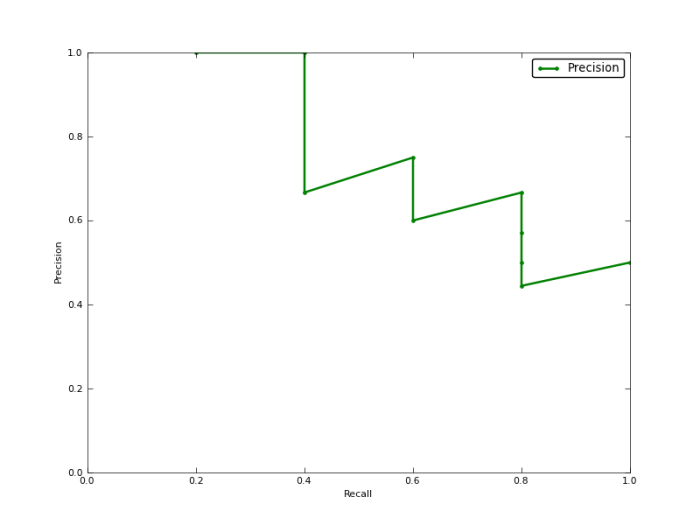### Average Precision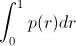That is equal to taking the area under the curve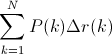=(1 * （0.2-0）) + (1 * (0.4-0.2)) + (0.66 * (0.4-0.4)) + (0.75 * (0.6-0.4)) + (0.6 * (0.6-0.6)) + (0.66 * (0.8-0.6)) + (0.57 * (0.8-0.8)) + (0.5 * (0.8-0.8)) + (0.44 * (0.8-0.8)) + (0.5 * (1-0.8)) = 0.782.

=(1 * 0.2) + (1 * 0.2) + (0.66 * 0) + (0.75 * 0.2) + (0.6 * 0) + (0.66 * 0.2) + (0.57 * 0) + (0.5 * 0) + (0.44 * 0) + (0.5 * 0.2) = 0.782.

### Interpolated average precision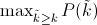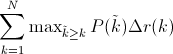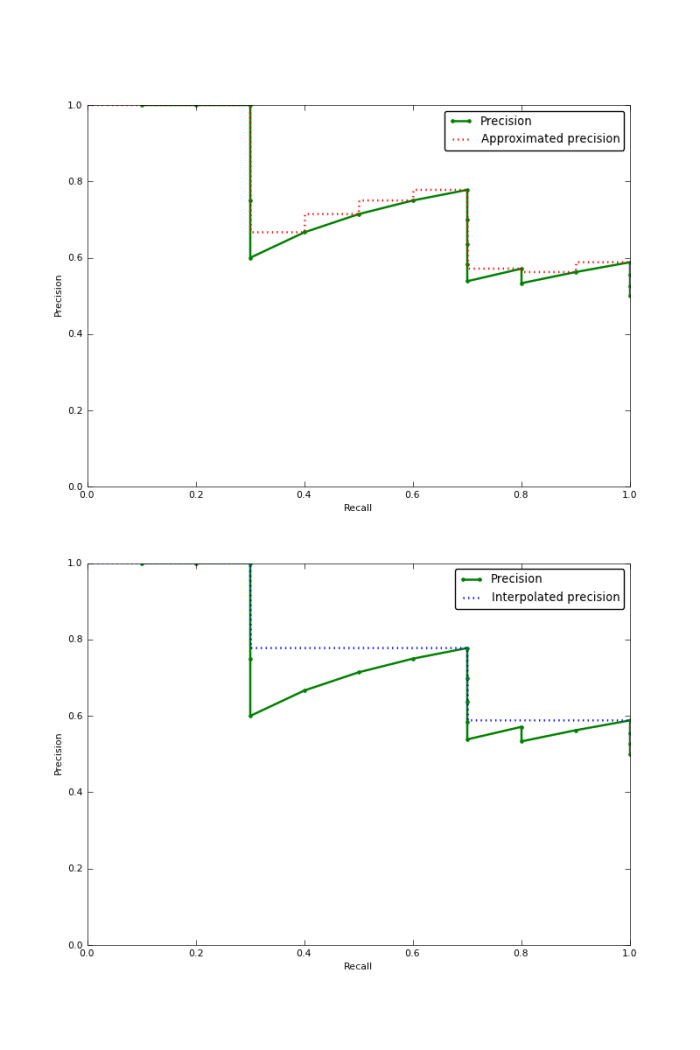### IoU

IoU这一值，可以理解为系统预测出来的框与原来图片中标记的框的重合程度。

IoU=DetectionResultGroundTruthDetectionResultGroundTruth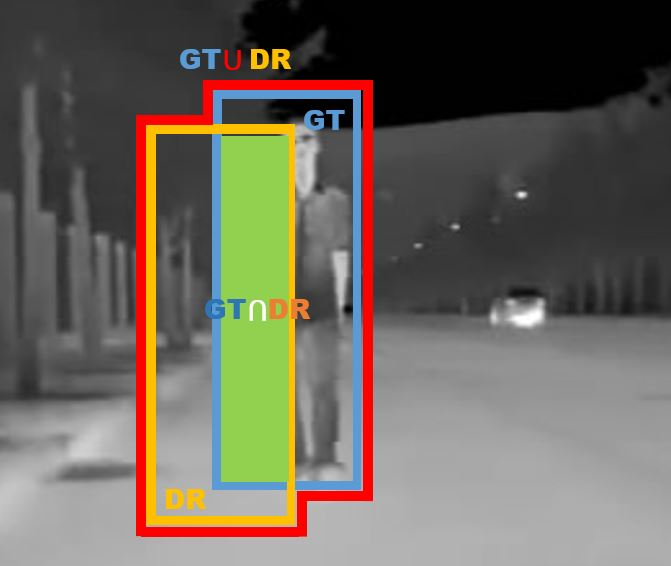2017年5月10日 19:07